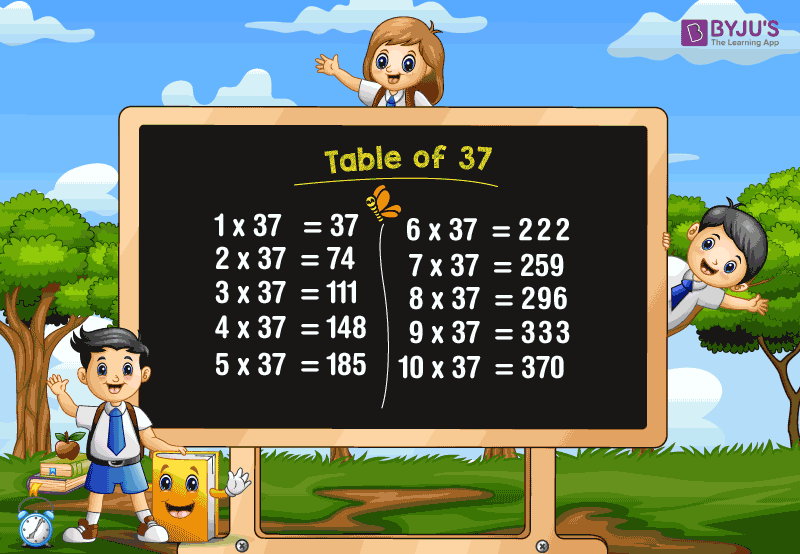# Table of 37

Table of 37, also called the 37 times table that shows the multiplication of the number 37 with different whole numbers. Learning the multiplication table of 37 is very much essential for every child, as it helps to do fast calculations. The multiplication tables enable the child to do complex calculations faster and in the easiest way. In this article, we will learn the multiplication table of 37 with the help of a chart and also, we are going to learn how to read the 37 times table.

### Download Table of 37 Here## 37 Times Table

To obtain the table of 37, we need to multiply 37 with the different whole numbers. If we repeatedly add the number 37, we get the table of 37, as the multiplication is the repeated addition process.

For example, 37 times 5 is 185.

(i.e) 37 × 5 = 185.

We will get the same answer if we repeatedly add the number 37 for 5 times.

(i.e.) 37+37+37+37+37 = 185.

Also, check tables from 1 to 100 for more details.

## Multiplication Table of 37

The multiplication table of 37 chart helps to learn the 37 times tables easily, and helps to solve the problem easily.

 37 × 1 = 37 37 × 2 = 74 37 × 3 = 111 37 × 4 = 148 37 × 5 = 185 37 × 6 = 222 37 × 7 = 259 37 × 8 = 296 37 × 9 = 333 37 × 10 = 370 37 × 11 = 407 37 × 12 = 444 37 × 13 = 481 37 × 14 = 518 37 × 15 = 555 37 × 16 = 592 37 × 17 = 629 37 × 18 = 666 37 × 19 = 703 37 × 20 = 740

### How to Read 37 Times Table?

Read the table of 37 as follows:

• One time thirty-seven is 37
• Two times thirty-seven is 74
• Three times thirty-seven is 111
• Four times thirty-seven is 148
• Five times thirty-seven is 185
• Six times thirty-seven is 222
• Seven times thirty-seven is 259
• Eight times thirty-seven is 296
• Nine times thirty-seven is 333
• Ten times thirty-seven is 370
• Eleven times thirty-seven is 407
• Twelve times thirty-seven is 444
• Thirteen times thirty-seven is 481
• Fourteen times thirty-seven is 518
• Fifteen times thirty-seven is 555.

Get More Maths Tables:

## Frequently Asked Questions on Table of 37

### 1. What is the table of 37?

Table of 37 is also called the 37 times table, which shows the multiples of 37. The multiplication table of 37 will be obtained if we multiply 37 with different whole numbers.

### 2. What is the value of 7 times 37?

7 times 37 is 259. (i.e.) 7 × 37 = 259.

### 3. Mention the first 10 multiples of 37.

The first 10 multiples of 37 are 37, 74, 111, 148, 185, 222, 259, 296, 333, and 370.

### 4. What is the value of 37 times 15?

37 times 15 is 555. (i.e.) 37×15 = 555.

### 5. What is the value of x, if 37x = 481?

Given, 37x = 481.
x=481/37
x=13
Hence, the value of x is 13.